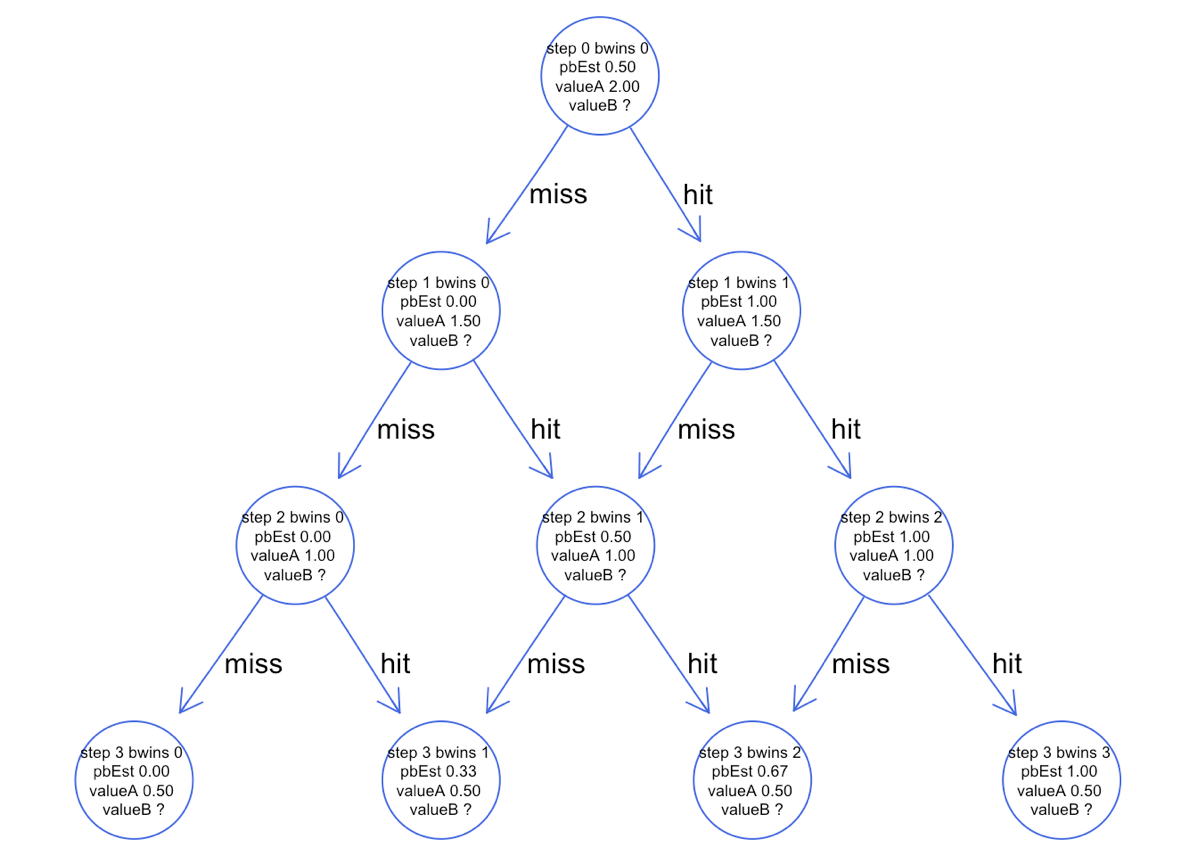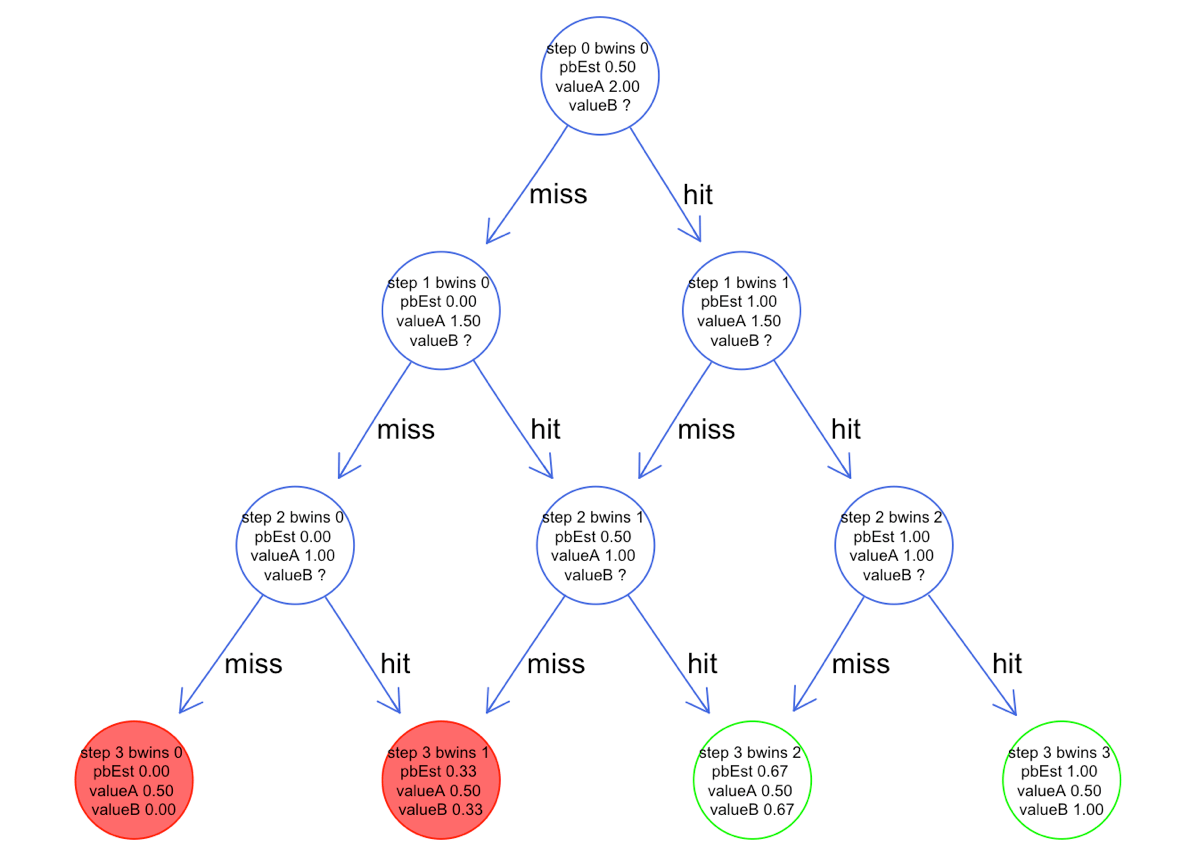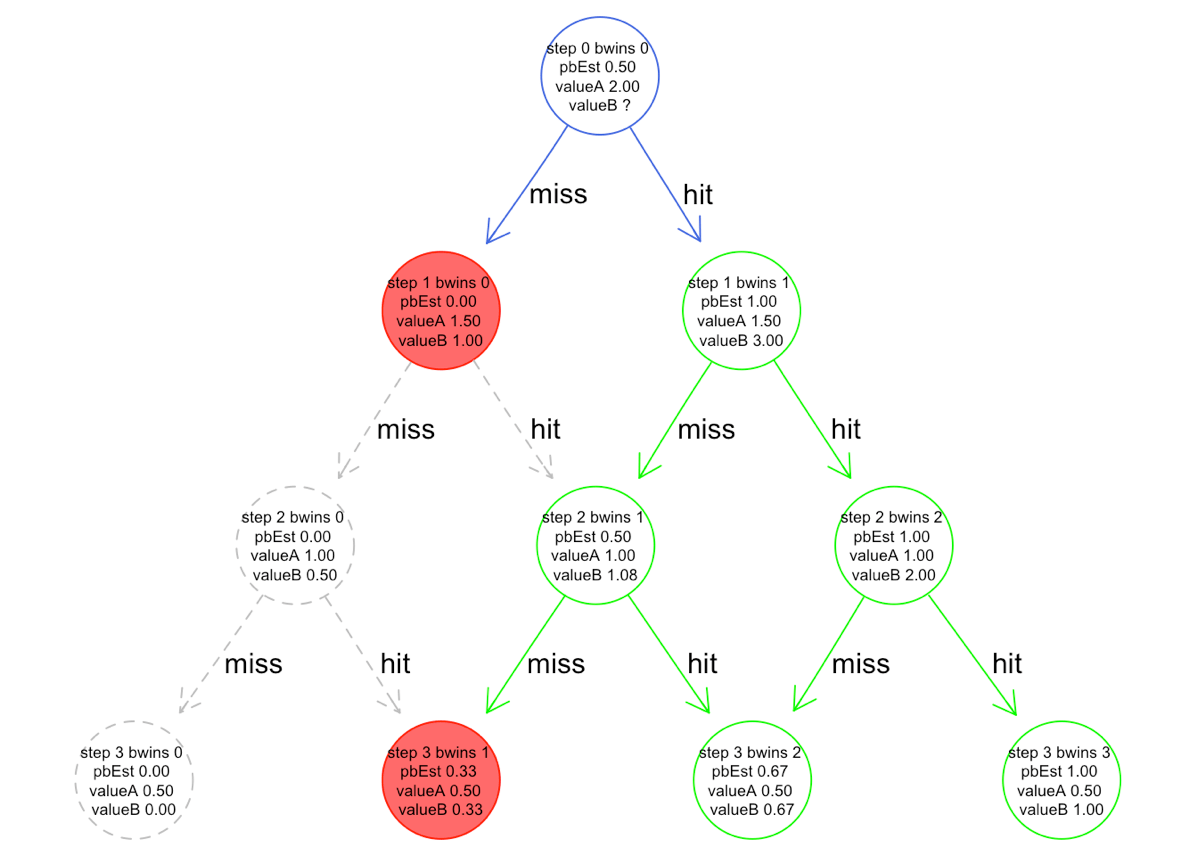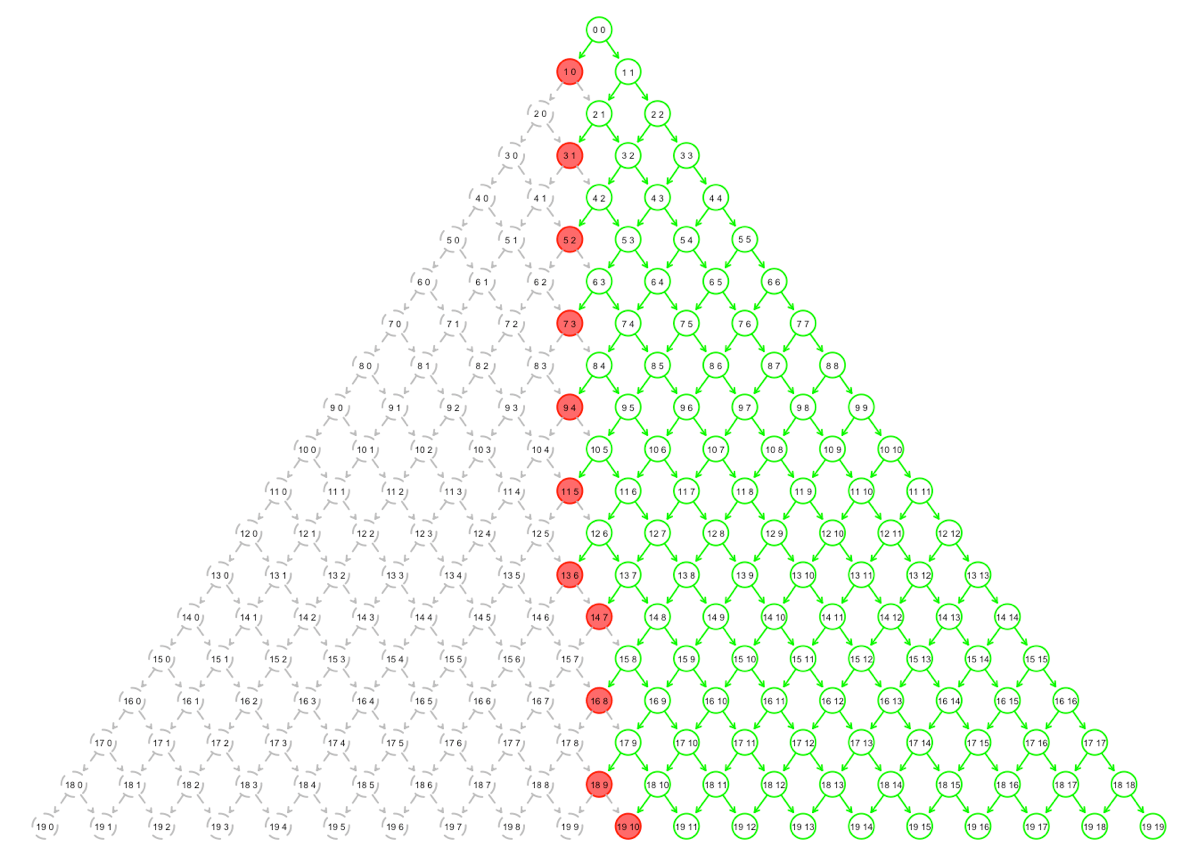# A dynamic programming solution to A/B test design

Our last article on A/B testing described the scope of the realistic circumstances of A/B testing in practice and gave links to different standard solutions. In this article we will be take an idealized specific situation allowing us to show a particularly beautiful solution to one very special type of A/B test.

For this article we are assigning two different advertising message to our potential customers. The first message, called “A”, we have been using a long time, and we have a very good estimate at what rate it generates sales (we are going to assume all sales are for exactly \$1, so all we are trying to estimate rates or probabilities). We have a new proposed advertising message, called “B”, and we wish to know does B convert traffic to sales at a higher rate than A?

We are assuming:

• We know exact rate of A events.
• We know exactly how long we are going to be in this business (how many potential customers we will ever attempt to message, or the total number of events we will ever process).
• The goal is to maximize expected revenue over the lifetime of the project.

As we wrote in our previous article: in practice you usually do not know the answers to the above questions. There is always uncertainty in the value of the A-group, you never know how long you are going to run the business (in terms of events or in terms of time, and you would also want to time-discount any far future revenue), and often you value things other than revenue (valuing knowing if B is greater than A, or even maximizing risk adjusted returns instead of gross returns). This represents severe idealization of the A/B testing problem, one that will let us solve the problem exactly using fairly simple R code. The solution comes from the theory of binomial option pricing (which is in turn related to Pascal’s triangle).Yang Hui (ca. 1238–1298) (Pascal’s) triangle, as depicted by the Chinese using rod numerals.

For this “statistics as it should be” (in partnership with Revolution Analytics) article let us work the problem (using R) pretending things are this simple.

## The problem

Abstractly we have two streams of events (“A” events and “B” events). Each event returns a success or a failure (say valued at \$1 and \$0, respectively)- and we want to maximize our overall success rate. The special feature of this problem formulation is that we assume we know how long we are going to run the business: there is an n so the total number of events routed to A (call this amount a) plus the total amount of events routed to B (call this b) is such that a+b=n.

To make things simple assume:

• There are no time varying factors (very unrealistic, dealing with time varying factors is one of the reasons you run A and B at the same time).
• All potential opportunities are considered identical and exchangeable.

The usual method of running an A/B test is to fix some parameters (prior distribution of expected values, acceptable error rate, acceptable range of error in dollars per event) and then design an experiment that estimates which of A or B is the most valuable event stream. After the experiment is over you then only work with whichever of A or B you have determined to be the better event stream. You essentially divide your work into an experimentation phase followed by an exploitation phase.

Suppose instead of deriving formal statistical estimates instead we solved the problem using ideas of operations research and asked for an adaptive strategy that directly maximized expected return rate? What would that even look like? It turns out you get a sensing procedure that routes all of its experiments to B for a while and then depending on observed returns may switch over to working only with A. This looks again like a sensing phase followed by an exploitation phase, but the exact behavior is determined by the algorithm interacting with experiment returns and is not something specified by the user. Let’s make things concrete by working a very specific example.

## A small example

For the sake of argument: suppose we are willing to work with exactly four events ever, A’s conversion rate is exactly 1/2, and we are going to use what I am calling “naive priors” on the rate B returns success. The entire task is to pick whether to work next with an event from A or from B. One strategy is a fill in of the following table:

Number of B-trials run Number of B-successes seen Decision to go to A or B next
0 0 ?
1 0 ?
1 1 ?
2 0 ?
2 1 ?
2 2 ?
3 0 ?
3 1 ?
3 2 ?
3 3 ?

Notice we have not recorded the number of times we have tried the A-event. Because we are assuming we know the exact expected value of A (in this case 1/2) there is an optimal strategy that never tries an A-event until the strategy decides to give up on B. So we only need to record how many B’s we have tried, how many successes we have seen from B, and if we are willing to go on with B. Remember we have exactly four events to route to A and B in combined total, and this is why we don’t need to know what decision to make after the fourth trial.

We can present the decision process more graphically as the following directed graph:Each row of the decision table is represented as node in the graph. Each node contains the following summary information:

• step: the number of B-trials we have run prior to this stage. In our procedure once we decide to run an A we in fact switch to A for the remaining trials (as we assumed we knew the A success rate perfectly we are assuming we can’t learn anything about A going forward, so we would have no reason to ever switch back).
• bwins: the number of successes we have seen from our B-trials prior to this stage.
• pbEst: the empirical estimate of the expected win-rate for this node. The idea is a node that has tried B n times and seen w wins should naively estimate the unknown true success rate of B as w/n (which is what is written in the node). A special case is the first node, which we started with 1/2 instead of 0/0.
• valueA: the value to be gained by switching over to the A events at this time. This is just how many events remain to process (four at the root node, and only 1 at the leaves) times our known value of A (1/2 in this case). Notice this is an expected value, we are not actually running the A’s and recording empirical frequencies; but instead multiplying the known expected value by the number remaining trials.
• valueB: the value to be gained by trying B one more time and then optimally continuing until the end of the 4 event trial (picking B’s or A’s for the remaining events as appropriate). Note that valueB ignores any payoff we have seen in getting to this node (that is already recorded in bwins and pbEst), it is only the value we expect from the remaining plays assuming our next draw is from B. If valueB>valueA then our optimal strategy is to go with B (and could fill in our earlier table with this decision). If we could just solve for valueB we would have our optimal strategy.
• (not shown): value: defined as max(valueA,valueB), the future value of a given node under the optimal strategy

The first two values (step,bwins) are essentially the keys that identify the node and the other fields (known or unknown) are derived values. In our example directed graph we have written down everything that is easy to derive (pbEst), but still don’t know the thing we want: valueB (or equivalently whether to try B one more time at each node).

It turns out there is an easy way to fill in all of the unknown valueB answers in this diagram. The idea is called dynamic programming and this application of it is inspired from something called the binomial options pricing model. But the idea is so simple yet powerful that we can actually just directly derive it for our problem.

Consider the leaf-nodes of the directed graph (the nodes with no exiting edges representing our the state of the world before our last decision). For these nodes we do have an estimate of valueB: pbEst! We can fill in this estimate to get the following refined diagram:For the final four nodes we know whether to try B again (the open green nodes) or if to give up on B and switch to A (the shaded red nodes). The decision is based on our stated goal: maximizing expected value. And in our last play we should go with B only if its estimated expected value is higher than the known expected value of A. Using the observed frequency of B-successes as our estimate of the probability of B (or expected value of B) may seem slightly bold in this context, but it is the standard way to infer (we can justify this either through Frequentist arguments or by Bayesian arguments using an appropriate beta prior distribution).

So we now know how to schedule the fourth and final stage. That wouldn’t seem to help us much: as the first decision (the top row, or root node) is what we need first, and it still has a “?” for valueB. But look at the three nodes in the third stage. We can now estimate their value using known values from the fourth stage.

Define:

• pbEst[step=n,bwins=w]: as the number written for pbEst in the node labeled “step n bwins w”.
• valueA[step=n,bwins=w]: as the number written for valueA in the node labeled “step n bwins w”.
• valueB[step=n,bwins=w]: as the number written for valueB in the node labeled “step n bwins w”.
• value[step=n,bwins=w]: max(valueA[step=n,bwins=w],valueB[step=n,bwins=w]).

The formula for valuing any non-leaf node in our diagram is:

``` valueB[step=n,bwins=w] = ( pbEst[step=n,bwins=w] * (1+value[step=n+1,bwins=w+1]) ) + ( (1-pbEst[step=n,bwins=w]) * value[step=n+1,bwins=w] ) ```

So if we know all of pbEst[step=n,bwins=w], value[step=n+1,bwins=w+1], and value[step=n+1,bwins=w]: we then know valueB[step=n,bwins=w]. This is just saying the valueB of a node is the value of the immediate next step if we played B (which gives us a bonus of 1 if B gives us a success) plus the value of the node we end up at.

For example we can calculate valueB of the “step 2 bwins 1” as:

``` valueB[step=2,bwins=1] = ( pbEst[step=2,bwins=1] * (1+value[step=3,bwins=2]) ) + ( (1-pbEst[step=2,bwins=1]) * value[step=3,bwins=1] ) = ( 0.5 * (1+0.67) ) + ( (1-0.5) * 0.5 ) = 1.085 ```

All this is just done by reading quantities off the current diagram. We can do this for all of the nodes in the third row yielding the following revision of the diagram.In the above diagram we have rendered nodes we consider unreachable (nodes we would never go to when following the optimal strategy) with dashed lines. We now have enough information in the diagram to use the equation to fill in the second row:And finally we fill in the first row (or root node) and have a complete copy of the optimal strategy.We can copy this from the diagram back to our original strategy table by writing “Choose A” or “Choose B” depending if valueA ≥ value B for the node corresponding to the line in the table.

Number of B-trials run Number of B-successes seen Decision to go to A or B next
0 0 Choose B
1 0 Choose A
1 1 Choose B
2 0 Choose A
2 1 Choose B
2 2 Choose B
3 0 Choose A
3 1 Choose A
3 2 Choose B
3 3 Choose B

### Pessimal priors for B

We don’t have to use what we have called naive estimates for pbEst. If we have good prior expectations on the likely success rate of B we can work this into our solution through the form of Bayesian beta priors. For example if our experience was such that the expected value of B is in fact around 0.25 (much worse than A) with a standard deviation of 0.25 (somewhat diffuse, so there is a non-negligible chance B could be better than A) we could design our pbEst calculation with that prior (which is easy to implement as a beta distribution with parameters alpha=0.5 and beta=1.5). In the case where we are only going to try A or B at total of four times we get the following diagram:This diagram is saying: for only four trials we already know enough to never try B. The optimal strategy is to stick with A all four times. However, if the total number of trials we were budgeted to run was larger (say 20) then it actually starts to make sense to try B a few times to see if it is in fact a higher rate than A (despite our negative prior belief). We demonstrate the optimal design for this pessimal prior and n=20 in the following diagram.And this is the magic of the dynamic programming solution. It uses the knowledge of how long you are going to run your business to decide how to value exploration (possibly losing money by giving traffic to B) versus exploitation (going with which of A or B is currently thought to be better). Notice the only part of the diagram or strategy table we need to keep is the list of nodes where we decide to no longer ever try B (the filled red stopping nodes). This is why we call this variation of the A/B test a stopping time problem.

All the B-rate calculations above are exactly correct if we in fact had the exact right priors for B’s rate. If the priors were correct at the root node, then by Bayes law the pBest probability estimates are in fact exactly correct posterior estimates at each node, and every decision made in the strategy is then correct. For convenience we have been using a beta distribution as our prior (as it has some justification, and makes calculation very easy), but these is no guarantee that the actual prior is in fact beta or that we even have the right beta distribution as our initial choice (the beta distribution is a determined by two parameters alpha and beta).

However, with n large enough (i.e. a budget of enough proposed events to design a good experiment) the strategy performance starts to become insensitive to the chosen prior (see the Bernstein–von Mises theorem for some motivation). So the strategy performs nearly as well with a prior we can supply as with the unknown perfect prior. As long as we start with an optimistic prior (one that allows our algorithm to route traffic to B for some time) we tend to do well.

## What if we do not know the exact expected value of A?

In practice we would never know the exact expected value of A (and certainly not know it prior to starting the experiment). In the more realistic situation where we assume we are trying to choose between an A and B where we have things to learn about both groups the dynamic programming solution still applies: we just get a larger dynamic programming table. Each state is indexed by four numbers:

1. nA: number of A trials already tried.
2. awins: number of A successes already seen.
3. nB: number of B trials already tried.
4. bwins: number of B successes already seen.

For each so-labeled state we have four derived values:

1. paEst: the estimate expected value of A in this state, this is a simple function of the state index label.
2. pbEst: the estimate expected value of B in this state, this is a simple function of the state index label.
3. valueA: the estimated expected continuation value of sending the next unit of traffic to A and then continuing on an optimal strategy. This starts out unknown.
4. valueB: the estimated expected continuation value of sending the next unit of traffic to B and then continuing on an optimal strategy. This starts out unknown.

And again, an optimal strategy is one that just chooses A or B depending on if valueA > valueB or not. Notice that in this case an optimal strategy may switch back and forth between using A or B experiments. The derived values are filled in from states at or near the end of the entire experiment just as before. We now have an index consisting of four numbers (nA,wA,nB,wB) instead of just two numbers (nB,wB) so it is harder to graphically present the intermediate calculations and the final strategy tables.

## A larger example

Here is an example that is closer to the success rates and length of business seen in email or web advertising (though one problem for email advertising is that this is sequential plan, we need all earlier results back to make later decisions). Suppose we are going to run an A/B campaign for a total of 10,000 units of traffic, we assume the A success rate is exactly 1%, and we will use the uninformative Jefferys prior for B (which is actually pretty generous to B as this prior has initial expected value 1/2). That is it: our entire problem specification is the assumed A-rate, the total amount of traffic to plan over, and the choice of B-prior. This is specific enough for the dynamic programming strategy to completely solve the problem of maximizing expected revenue.

The dynamic program solution to this problem can be concisely represented by the following graph:The plan is: we route all traffic to B, always measuring the empirical return rate of B (number of B successes over number of B trials). The number of B trials is the x-axis of our graph and we can use the current estimated B return rate as our y-height. The decision is: if you end up in the red area (below the curve) you stop B and switch over to A forever. Notice B is initially given a lot of leeway. It can fail to pay off a few hundred times and we don’t insist on it having a success rate near A’s 1% until well over 5,000 trials have passed.

## Conclusion

Dynamic programming offers an interesting alternative solution to A/B test planning (in contrast to the classic methods we outlined here).

All the solutions and diagrams were produced by R code we share here.

## Next

We will switch from “statistics as it should be” back to “R as it is” and discuss the best ways to incrementally collect data or results in R.### jmount

Data Scientist and trainer at Win Vector LLC. One of the authors of Practical Data Science with R.

### 2 replies ›

1.shankar Prawesh (@sprawesh) says:

Nice example of using option pricing technique for A/B testing

2.jmount says:

I’ve seen some questions about the Gittins index. Basically at some point you want to use the reasoning of the dynamic programming solution, but avoid all of the bookkeeping. You do this by introducing a few ideas (such as using large time-scale quantity such the Gittins index as an approximation for the node values, and introducing policy iteration to approximate solutions).

Nina had a great article on exploring the trade-offs of bandit formulations earlier Bandit formulations for A/B tests some intuition (in particular the graphs she produced tell you a lot about the trade-off of doing well assuming B is better versus doing well assuming B is worse).

One of the fun observations is the dynamic programming solution gives you an example of an alternate form of reasoning over uncertainty that doesn’t build intervals or estimate variances. This makes it easier to explain that things like confidence intervals or things like credible intervals are emergent constructs we introduce to think about probabilistic systems, and not necessarily axiomatic or primary concepts.

When we get back to the A/B testing topic (which probably will be a while) we will write about continuous inspection tables (such as those found Wald’s Sequential Analysis). These treatments really wrap the whole thing up quite nicely.